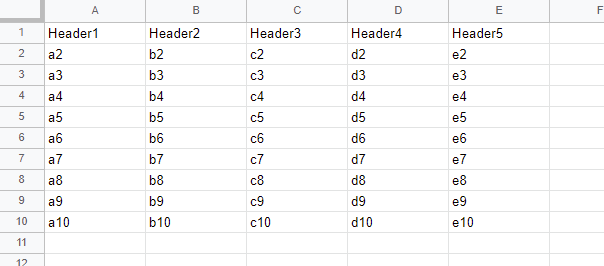Gists

## Introduction

In this report, I would like to introduce to apply the spread syntax and the destructuring assignment to Google Spreadsheet with Google Apps Script. The destructuring assignment can be used without V8 runtime. But, the spread syntax is required to be used with V8 runtime. Recently, I often saw the script using them at Stackoverflow. And also, I have sometimes gotten the questions related to the spread syntax and the destructuring assignment. So, I thought that I would like to introduce in my blog.

In generally, the data structure of most Spreadsheets is the header row in the 1st row and the data rows below 2nd row showing in the following image.## Sample situation 1

As the sample situation 1, when the values are retrieved from the such the data structure using Google Apps Script, in most cases, the following script might have used.

const sheet = SpreadsheetApp.getActiveSheet();
const values = sheet.getRange("A2:E" + sheet.getLastRow()).getValues();


and

const sheet = SpreadsheetApp.getActiveSheet();
const data = sheet.getDataRange().getValues();
const values = data;


In this case, when the above sample Spreadsheet is used, header and values are as follows, respectively.

["Header1", "Header2", "Header3", "Header4", "Header5"]

[
["a2", "b2", "c2", "d2", "e2"],
["a3", "b3", "c3", "d3", "e3"],
["a4", "b4", "c4", "d4", "e4"],
["a5", "b5", "c5", "d5", "e5"],
["a6", "b6", "c6", "d6", "e6"],
["a7", "b7", "c7", "d7", "e7"],
["a8", "b8", "c8", "d8", "e8"],
["a9", "b9", "c9", "d9", "e9"],
["a10", "b10", "c10", "d10", "e10"]
]


Here, when the spread syntax and the destructuring assignment are used for this situation, the script becomes as follows.

const sheet = SpreadsheetApp.getActiveSheet();


In this case, the script becomes simple. The result values of header and values are the same with the above one.

### Explanation

sheet.getDataRange().getValues() returns the following values.

[
["a2", "b2", "c2", "d2", "e2"],
["a3", "b3", "c3", "d3", "e3"],
["a4", "b4", "c4", "d4", "e4"],
["a5", "b5", "c5", "d5", "e5"],
["a6", "b6", "c6", "d6", "e6"],
["a7", "b7", "c7", "d7", "e7"],
["a8", "b8", "c8", "d8", "e8"],
["a9", "b9", "c9", "d9", "e9"],
["a10", "b10", "c10", "d10", "e10"]
]


... of ...values is the spread syntax. header and ...values of [header, ...values] mean 1st row and the rows below 2nd rows. For example, when const [header, values] = sheet.getDataRange().getValues() is run, header and values return 1st row and 2nd row, respectively. From this situation, it is found that ... of ...values means the multiple rows. By this, the above result is obtained.

## Sample situation 2

As the sample situation 2, there is a case that the specific columns have to be retrieved from Spreadsheet. For example, it thinks of the case that the columns “B” and “D” are retrieved from the above sample Spreadsheet. In this case, by the destructuring assignment, the simple sample script can be used.

const sheet = SpreadsheetApp.getActiveSheet();
const values = sheet.getDataRange().getValues();
const res = values.map(([a, b, c, d, e]) => [b, d]);


When this script is run to the above Spreadsheet, the following result is obtained.

[
["b2", "d2"],
["b3", "d3"],
["b4", "d4"],
["b5", "d5"],
["b6", "d6"],
["b7", "d7"],
["b8", "d8"],
["b9", "d9"],
["b10", "d10"]
]


### Explanation

For example, when const res = values.map((r) => r) is run, the result of res is the same with values. Namely, r of values.map((r) => r) is each row.

Here, [a, b, c, d, e] of values.map(([a, b, c, d, e]) => [b, d]) means the columns “A” to “E”. By this, you can easily select the specific columns like [b, d].

In the above situation, only the columns “B” and “D” are retrieved. So the above script can be also modified as follows.

const sheet = SpreadsheetApp.getActiveSheet();
const values = sheet.getDataRange().getValues();
const res = values.map(([, b, , d]) => [b, d]);


Of course, you can use const res = values.map((r) => [r, r]). But from the benchmark, the process cost for using the destructuring assignment is lower than that for using the index.

But, when the number of column is large, when the destructuring assignment is used, the readability of script might be low. In that case, using index is suitable. So, please be careful about this.

## Note

I think that the above methods can be also used for various situations except for Spreadsheet. So when this post was useful for your situation, I’m glad.